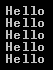Support us .Net Basics C# SQL ASP.NET Aarvi MVC Slides C# Programs Subscribe Download

### Part 28 - Generation Operators in LINQ

Suggested Videos
Part 25 - Cross Join in LINQ
Part 26 - Set operators in LINQ
Part 27 - Union, Intersect and Except operators in LINQ

The following operators belong to Generation Operators category
Range
Repeat
Empty

Range operator generates a sequence of integers within a specified range. This method has 2 integer parameters. The start parameter specifies the integer to start with and the count parameter specifies the number of sequential integers to generate.

For example to print the first 10 even numbers without using LINQ, we would use a for loop as shown below.
for (int i = 1; i <= 10; i++)
{
if (i % 2 == 0)
{
Console.WriteLine(i);
}
}

To achieve the same using LINQ, we can use Range method as shown below.

var evenNumbers = Enumerable.Range(1, 10).Where(x => x % 2 == 0);

foreach (int i in evenNumbers)
{
Console.WriteLine(i);
}

Output :Repeat operator is used to generate a sequence that contains one repeated value.

For example the following code returns a string sequence that contains 5 "Hello" string objects in it.
var result = Enumerable.Repeat("Hello", 5);

foreach (var v in result)
{
Console.WriteLine(v);
}

Output:Empty operator returns an empty sequence of the specified type. For example
Enumerable.Empty<int>() - Returns an empty IEnumerable<int>
Enumerable.Empty<string>() - Returns an empty IEnumerable<string>

The question that comes to our mind is, what is the use of Empty() method. Here is an example where we could use Empty() method

There may be scenarios where our application calls a method in a third party application that returns IEnumerable<int>. There may be a situation where the third party method returns null. For the purpose of this example, let us assume the third party method is similar to GetIntegerSequence().

A NULL reference exception will be thrown if we run the following code
class Program
{
public static void Main()
{
IEnumerable<int> result = GetIntegerSequence();

foreach (var v in result)
{
Console.WriteLine(v);
}
}

private static IEnumerable<int> GetIntegerSequence()
{
return null;
}
}

One way to fix this is to check for NULL before looping thru the items in the result as shown below.
class Program
{
public static void Main()
{
IEnumerable<int> result = GetIntegerSequence();

if (result != null)
{
foreach (var v in result)
{
Console.WriteLine(v);
}
}
}

private static IEnumerable<int> GetIntegerSequence()
{
return null;
}
}

The other way to fix it, is by using Empty() linq method as shown below. Here we are using NULL-COALESCING operator that checks if the GetIntegerSequence() method returns NULL, in which case the result variable is initialized with an empty IEnumerable<int>.
class Program
{
public static void Main()
{
IEnumerable<int> result = GetIntegerSequence() ?? Enumerable.Empty<int>();

foreach (var v in result)
{
Console.WriteLine(v);
}
}

private static IEnumerable<int> GetIntegerSequence()
{
return null;
}
}